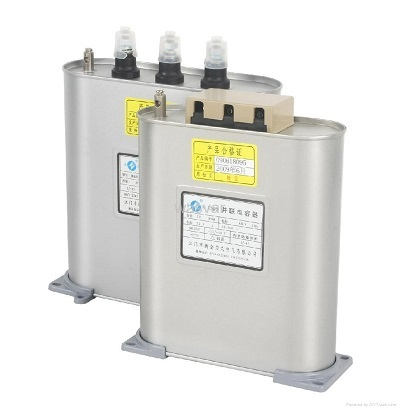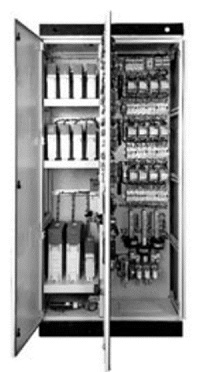# Capacitors – Get More Power & Save Money

Power factor is the ratio between the KW and the KVA drawn by an electrical load. Where the KW is real power to load and KVA is the apparent load power. It is the measurement how an electrical equipment getting current in actual. A load with power factor of 0.85, results much higher losses in supply system and consumer has to pay higher bill… - Anuraj KumarIndustrial Consumer has to pay to utilities for the power that he is not using. The power we connect at load has mixed of real power and apparent power, we can use only real power to run our equipment but bill is charged on total power but there are means by which Kilowatt hours can be reduced slightly and that is done by improving power factor.

Power Factor

Let’s understand power factor first, it is the ratio between the KW and the KVA drawn by an electrical load. Where the KW is real power to load and KVA is the apparent load power. It is the measurement how an electrical equipment getting current in actual. A load with power factor of 0.85, results much higher losses in supply system and consumer has to pay higher bill. Little improvement in power factor lead to significant reduction in losses since losses are proportional to square of the current.When power factor is less than one the ‘lost power’ is known as reactive power or you can call it waste power. A poor power factor is result of phase difference between voltage and current, generally inductive load like induction motor, power transformer, induction furnace is main cause for poor power factor.

Causes of poor Power factor

Main causes of poor power factor is inductive load, most common ones are;

1. Single phase and three phase induction motor, usually induction motor works at poor power factor i.e full load pf=0.8-0.9,small load pf =0.2-0.3,no load pf =0
2. Varying load in power system, as we know that load on power system is varying. During low load period, supply voltage is increased, which increase the magnetising current, which causes the decreased power factor
3. Industrial heating furnaces
4. Welding machines
5. Electrical discharge lamps
6. Transformer
7. Harmonic currentsCorrecting the power factor

Inductive load require a magnetic field to operate and while creating such magnetic load current lags the voltage. We need to compensate these lagging current by leading current and this is done by capacitors.

Power factor correction is done by connecting the capacitors in parallel in motor circuit. The resulting capacitive current is leading current and it cancels the lagging inductive current flowing from the supply.By adding capacitor in circuit to correct power factor, we can get benefits;

• Minimise reactive losses in system
• Generate torque in single phase motor
• Prevent self – excitation in asynchronous motor
• Limit harmonics in system

Capacitors types

The dielectric material determines the type of capacitor. Common types of capacitors are;

• MICA capacitors: used as high frequency and telecommunication capacitors.
• CERAMIC capacitors: used in telecommunication applications when there is not enough space.
• ELECTROLYTIC capacitors: used mainly for direct current rectifications.
• TRIM capacitors: their capacitance can be modified depending on the needs of the application.

Capacitors Selection for power factor compensation:

To identify the requirement of a capacitor you require following details:1. Type : All poly propylene ( APP) , Metallised polypropylene (MPP) , Mix Dielectric,
2. Box , Can type body
• Inductive (Motor), Resistive (Lighting), etc.